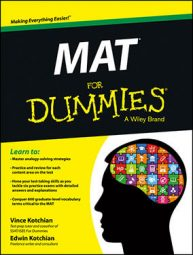##### MAT For DummiesMass, velocity, acceleration — yep, it’s time to review all the physics you learned in high school and prepare for the MAT (Miller Analogies Test). For the MAT, getting familiar with physics doesn’t entail learning any complicated equations. You just have to remember some physics terms and learn about some famous physicists. These lists help you remember the basics.

## Physics terms that appear on the MAT test

Brush up on your physics by studying these terms and their definitions.

• Acceleration: Rate at which velocity changes with time

• Acoustics: Science that deals with the study of mechanical waves

• Adhesion: Tendency for different types of particles to cling together

• Aerodynamics: Study of the motion of air when it interacts with solid objects

• Alloy: Metallic mixture of two different elements

• Alternating current: Movement of electric charge that reverses direction

• Ampere: Unit of electric current

• Angstrom: One ten-billionth of a meter

• Astronomical unit: Distance from Earth to the sun

• Background radiation: Ionizing radiation that is always present in Earth’s atmosphere

• Big bang: Model that explains the early development of the universe

• Binary star: Star system with two stars orbiting around each other

• Black hole: Region of space where gravity prevents everything from escaping

• Boson: Fundamental particle

• Buoyancy: Upward force that opposes the weight of a submerged object

• Celsius: Scale and measurement unit for temperature

• Centripetal force: Force that makes something follow a curved path

• Cohesion: Tendency for like particles to cling together

• Density: Mass per volume

• Dew point: Temperature at which water vapor condenses to liquid water

• Displacement: Fluid moving out of the way when an object is placed in it

• Doppler effect: Change in the frequency of a wave when the observer moves

• Drag: Air resistance or fluid resistance

• Elasticity: Property of an object returning to its original shape after a force is applied

• Endothermic: Reaction in which the system absorbs energy from its surroundings

• Equilibrium: A system in balance

• Exothermic: Reaction in which the system releases energy

• Fission: Process in which the nucleus of an atom splits into smaller parts

• Force: Mass times acceleration

• Friction: Force resisting the motion of materials sliding against each other

• Fusion: Process in which multiple atomic nuclei join to create a larger nucleus

• Gamma ray: Electromagnetic radiation of high frequency

• General relativity: Einstein’s theory of gravitation

• Hertz: Unit of frequency, defined as cycles per second

• Inertia: Resistance of an object to change in its motion

• Joule: Unit of heat

• Lever: Simple machine consisting of a beam pivoting on a hinge

• Light: Electromagnetic radiation visible to the human eye

• Mass: Amount of matter in a body

• Matter: Anything that has mass and volume

• Momentum: Mass times velocity

• Neutrino: Neutral elementary particle

• Ohm: Unit of electrical resistance

• Pendulum: Suspended weight that can swing on a pivot

• Photon: Elementary particle of light

• Pneumatics: Application of pressurized gas to affect motion

• Pressure: Ratio of force to area

• Pulley: Simple machine; a wheel on an axle that moves a cable along its circumference

• Quantum mechanics: Physics dealing with atomic and subatomic particles

• Supernova: Explosion of a star

• Temperature: Physical property that expresses notions of hot and cold

• Torque: Tendency of a force to rotate an object on an axis

• Transducer: Device that converts energy from one form to another

• Uncertainty principle: Principle that the position and momentum of a particle cannot be simultaneously known

• Vacuum: Space that is empty of matter

• Watt: Unit of power

## Important figures in the field of physics

The following lists important people in the field of physics you should become familiar with before taking the MAT.

• Ampere, Andre-Marie: French founder of classical electromagnetism

• Archimedes: Ancient Greek scientist known for hydrostatics

• Becquerel, Antoine Henri: French physicist who helped discover radioactivity

• Bernoulli, Daniel: Swiss physicist who worked on fluid dynamics

• Bohr, Niels: Danish physicist who contributed to quantum mechanics

• Einstein, Albert: German physicist known for general and special relativity

• Faraday, Michael: English scientist known for work in electromagnetism

• Fermi, Enrico: Italian-American physicist who developed the nuclear reactor

• Galileo: Italian astronomer and father of modern physics

• Heisenberg, Werner: German physicist credited for creating quantum mechanics

• Newton, Isaac: English physicist who described three laws of motion

• Planck, Max: German physicist who came up with quantum theory

• Tesla, Nikola: Serbian-American known for contributions to alternating current electricity